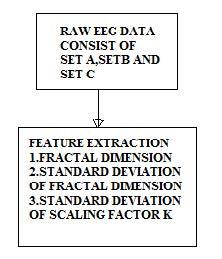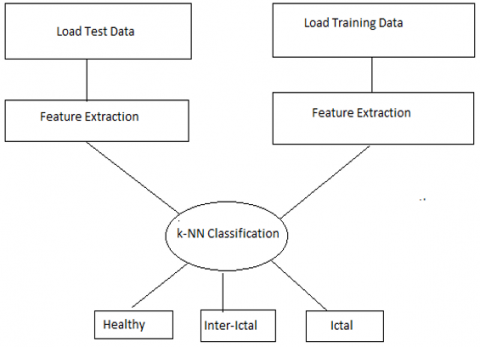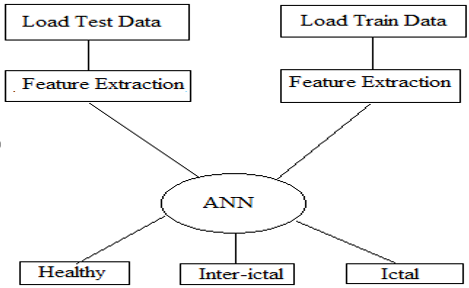# Classification of healthy, inter-ictal and seizure signal using various classification techniques

Classification of healthy, inter-ictal and seizure signal using various classification techniques

Hemant ChoubeyAlpana Pandey

Department of Electronics and Communication, MANIT-Bhopal, India

Corresponding Author Email:
hemantchoubey271986@gmail.com
Page:
75-84
|
DOI:
https://doi.org/10.3166/TS.35.75-84
|
Accepted:
|
Published:
30 April 2018
| Citation

OPEN ACCESS

Abstract:

Electroencephalography (EEG) is the study of brain electrical activity for different physiological state of the brain. The main purpose of this paper is to identify epileptical seizure signal from EEG signal through combinational classifier. Combinational classifier combine k-NN classifier with ANN classifier. k-NN classifier for detection of Healthy and Inter-ictal signal then ANN classifier for the detection of Seizure signal. These three classifier works on same input values of Fractal Dimension called as Higuchi Fractal Dimension. Higuchi Fractal Dimension derived three parameters (1) Fractal Dimension, (2) Standard Deviation of Fractal Dimension and (3) Standard Deviation of scaling factor used as inputs of the k-NN classifier, ANN classifier and Combinational Classifier respectively for the classification of EEG signal and Detection of Seizure signal from EEG signal. The findings of this research on the basis of this three input statistical parameter value performance parameter like Accuracy, Sensitivity and Selectivity calculated as 92.66%, 89% and 89% respectively and compared its performance with different statistical feature using other classifier.

Keywords:

electroencephalogram (EEG) signal, levenberg marquardt (LM) classifier, epileptic seizure detection, k-nearest neighbour (kNN), artificial neural network (ANN), and variance

1. Introduction

Electroencephalogram (EEG) signal is one of the extensively used research field for the diagnosis of Epileptic Seizure because the characteristic behavior of Epileptic signal is different from the Healthy EEG signal. In a worldwide, approximately 50 million people has suffered from neuronal chronic disease called as Epilepsy with unpredictable seizure signal. Mostly seizure prediction algorithm divides into three parts (a) Processing of EEG signal like filtering before Extraction of statistical parameter (b) Extraction of statistical parameters (c) Classification based on above parameter using linear and non-linear classifier. Related work of various seizure prediction algorithm depicted in section 1.1.

1.1. Related works

Wang et al., (2015) used Independent Component Analysis with Multivariate Empirical Mode Decomposition for the removal of electrooculography (EOG) artifacts from multichannel EEG signal as preprocessing of EEG data. This improves signal to noise ratio and decrease mean square error.

Pachori & Sircar, (2008) used a time order representation of EEG signal which can be useful for the analysis of multicomponent non-stationary signal. Patel et al. (2016) decomposed contaminated EEG signal into its IMF with correlation removed eye blink artifacts from EEG signal. Sharmila and Geethanjali (2016) used DWT technique for identifying the epileptic seizure from the EEG signals. This paper works on Naïve Bayes (NB) classifier with K-NN classification techniques for seizure detection. Here, the K-NN technique was utilized to identify the basic similarity between the training and testing sets. Then, the values of each attribute were normalized in order to provide a reduced error rate. The advantages that obtained from this work were, better accuracy, and reduced computation time. Tibdewal et al. (2016) analyzed a EEG signal through stationary wavelet transform. Four statistical features from stationary wavelet transform (Min, Max,Variance and Energy) shows significant difference from Epileptic and Non-Epileptic EEG signal.

From the survey, it is analyzed that the existing signal processing techniques has following drawbacks:

- Number of features used for classification of EEG signal is very large.

- Not able to classify three class of EEG signal i.e. Healthy, Inter-ictal and Seizure signal.

- It was highly dependent on the recorded length

To solve these issues, this paper intends to develop an combinational classification technique for detecting the epileptic seizure and classification of EEG signal into three set of class called as class A, class B and class C. Class A for Healthy signal, Class B for Inter-ictal signal and Class C for Ictal signal or Epileptic signal .In this paper two classifier are used, one is k-NN classifier for the classification of Healthy and Inter-ictal signal and other is ANN classifier for the detection of Seizure signal. A combination of k-NN and ANN classifier called as Combinational classifier shows results in this paper effectively using only HFD parameter. Higuchi Fractal Dimension of Satapathy et al paper for the classification of EEG signal works on the dimension level of EEG signal as a input of three types of classifier. The main objective of this paper depicted in section 1.2.

1.2. Objectives

The research objectives of this paper are as follows:

- To extract the features of Higuchi Fractal Dimension.

- To classify whether the signal is Healthy and Inter-ictal using K-Nearest Neighbor (KNN) and then ANN classification technique is implemented for the detection of Epilepsy. The rest of the section of this paper divides into five parts. Section 2 describes statistical parameter while Section 3 discuss feature extraction for the classification of EEG signal. Section 4 describes proposed technique of combinational classifier(KNN+ANN) with HFD parameter. Section 5 shows results and performance parameter using kNN,ANN and Combinational Classifier. Lastly Conclusion and Future Work discuss in Section 6 (Higuchi, 2016; Şeker & Özerdem, 2016).

2. Statistical parameter

2.1. Higuchi fractal dimension

It is a non-linear method in which Geometrical shape at different scale is the feature input used for the classification presented in the paper of Satapathy et al (2016) (Petrosian, 1995). According to this paper, HFD parameters can be extracted using different methods suggested by Petrosian(7) and Higuchi(8) and named accordingly Petrosian Fractal Dimension(PFD) and Higuchi Fractal Dimension(HFD)(9). PFD can be calculated as (1).

$P F D=\frac{\log _{10}(S)}{\log _{10}(S)+\log _{10}\left(\frac{S}{S+0.4 S}\right)}$         (1)

where S is the series length and Sψis the number of sign changes in the signal and HFD is the slope of line that best fits the curve of ln((Xmean(k)) and ln(1/k) defined in equation as(2)

$X_{\text {mean}}=\frac{\sum_{m=1}^{k} X(m, k)}{k}$        (2)

where

$X(m, k)=\frac{\sum_{i=2}^{\lfloor(N-m) / k\rfloor}\left\lfloor x_{m+i k}-x_{m+(i-1) k}\right\rfloor(N-1)}{\lfloor(N-m) / k\rfloor k}$

Three scaling features required for classifications are (1) Fractal Dimension FD that measures the complexity of series (2) SFD is the standard deviation of fractal dimension and (3) Sb is the standard deviation of scaling factor in which k is the hopping interval of signal. These feature values as input of the k-NN,ANN and Combinational classifier.

## 1.jpgFigure 1. Block diagram for HFD parameters

Figure 1 represent features required as input for classification of Healthy, Inter-ictal and ictal (Seizure) signal.

Algorithm for HFD calculation

Input: Input EEG signal;

Output: Signal Features of HFD;

Step I: Generate discrete time sequence x(i)  for i=1 to 4096.

Step II: Construct a new time series xmk from x(i) where m is  the initial time and k is the hopping interval of the  signal x(i) for m=1 to k as(3)

$x_{m k}=\left\{x(m), x(m+k), \ldots \ldots \mathrm{x}\left(\mathrm{m}+\mathrm{int}\left(\frac{N-m}{k}\right) k\right\}\right.$         (3)

Step III: Calculate the length of X(m,k) for each xmk can be calculated as(4)

$X(m, k)=\frac{1}{k}\left[\left(\sum_{i=1}^{\left(\frac{N-m}{k}\right)}|x(m+i . k)-x(m+(i-1) \cdot k)|\right)\right] *\left(\frac{N-1}{\ln t\left(\frac{N-m}{k}\right) \cdot k}\right)$        (4)

Step IV: Mean value of X(m,k) can be calculated as(5)

$X_{\text {mean}}=\frac{\sum_{m=1}^{k} X(m, k)}{k}$       (5)

Step V: Fractal Dimension FD can be calculated by a least-squares fitting procedure as

Y=aX+b

where a=FD according to the formula as(6)

$F D=\frac{n \sum\left(x_{k} \cdot y_{k}\right)-\Sigma x_{k} \Sigma y_{k}}{n \sum x_{k}^{2}-\left(\sum x_{k}\right)^{2}}$     (6)

where yk=ln(Xmean) and xk=ln(1/k) for k=1,2,3……kmax n denotes number of k values for which linear regression is calculated ($2 \leq n \leq k_{\max }$).

Step VI: Set kmax=9.

Step VII: The standard deviation of Fractal Dimension SFD can be calculated as (7).

$\mathrm{SFD}=\sqrt{\frac{n \cdot\left[\sum y_{k}^{2}-F D \sum x_{k} y_{k}-b \cdot \Sigma y_{k}\right]}{(n-2) \cdot\left[n \cdot \Sigma x_{k}^{2}-\left(\sum x_{k}\right)^{2}\right]}}$         (7)

where

with standard deviation of scaling factor k as (8).

$\mathrm{S}_{\mathrm{b}}=\sqrt{\frac{1}{n} \cdot S F D^{2} \cdot \sum x_{k}^{2}}$     (8)

2.2. Dataset description

EEG signals that obtained from this dataset which has five sets such as Set A, Set B, Set C, Set D, and Set E, which are obtained based on the followings:

Set A + Set B – It consist of Healthy EEG signal with eyes open and close respectively

Set C+ Set D – In this set, the EEG is recorded during the seizure free interval of hippocampal formation of the hemisphere of the brain (i.e. inter-ictal).

Set E – In this set, the EEG is recorded during the seizure activity (i.e. ictal).

3. Feature extraction and classification

After getting the input EEG signal, the features such as FD,SFD and Sb  of the test and train sample data extracted and used for the classification using k-NN and ANN classifier as shown in fig(2) and (3).

## 2.pngFigure 2. k-NN classification

## 3.pngFigure 3. ANN Classification

4. Proposed technique

In a proposed technique combinational classifier are used consist of k-NN classifier for Healthy and Inter-ictal signal while ANN classifier for Seizure signal with trainlm function of learning rate of 0.01 as shown in fig(4).Fig(4) shows EEG data provided by University of Bonn ,Germany as input for the extraction of statistical parameter. k- NN classifier based on the nearest neighbor distance is best suitable for the classification of Healthy signal and Inter-ictal (signal before seizure attack).While ANN classifier is used for the detection of seizure signal at the end with reduced number of statistical features like FD,SFD and Sb for the classification of EEG signal.

## 4.pngFigure 4. Combinational classifier for detection of epilepsy

5. Results and performance parameter

5.1. Accuracy

It is the ratio of total number of correct classification to the total number of the applied data segment as (9).

Accuracy $(\text { in } \%)=\frac{T P+T N}{T P+T N+F P N N} \times 100$        (9)

Selectivity

It is the false rejection of the ictal segments as (10)

Selectivity $(\text { in } \%)=\frac{T P}{T P+F P} \times 100$          (10)

Sensitivity

Sensitivity is the detection of ictal segment from given EEG sample data as (11)

Selectivity $(\text { in } \%)=\frac{T P}{T P+F P} \times 100$         (11)

Specificity

Specificity is the detection of Inter-ictal Segment as (12)

Specificity $(\text { in } \%)=\frac{T N}{T N+F P} \times 100$           (12)

Average Detection Rate

ADR is the average of Sensitivity and Selectivity as (13)

$\mathrm{ADR}=\frac{\text { Sensitivity }+\text { Specificity }}{2}$          (13)

Table 1. Classification using HFD parameter for k-NN and ANN classifier and combinational classifier

 k-NN Based Classification ANN Based  Classification Combinational Classifier Training percent=80% Training percent=80%    Learning rate=0.01 Training percent=80%    Learning rate=0.01 Matrix Matrix Matrix Healthy Inter-ictal Ictal Healthy Inter-ictal Ictal Healthy Inter-ictal Ictal Healthy 98 1 1 Healthy 89 3 8 Healthy 97 1 2 Inter-ictal 2 96 2 Inter-ictal 12 84 4 Inter-ictal 2 90 8 Ictal 2 1 97 Ictal 6 9 85 Ictal 13 7 80 Accuracy=98 Accuracy=90.66 Accuracy=92.667 Selectivity=97.01 Selectivity=86.10 Selectivity=89.11 Sensitivity==97 Sensitivity=86 Sensitivity=89 Specificity=67.0068 Specificity=68.38 Specificity=68.01 Average Detection Rate=82.0034 Average Detection Rate=77.19 Average Detection Rate=78.50

## 5.pngFigure 5. Performance plot

Table 1 shows classifier output with HFD parameter. This shows performance parameter like Accuracy 98% for the classification of Healthy and Inter-ictal signal while ANN classifier is suitable for the detection of Seizure signal with Accuracy of about 90% with computation time of 1sec. For Combinational Classifier, Accuracy becomes 92% with the same computational time and it will improve further with other statistical parameters for same training percentage. Fig5 shows performance plot like Mean square error with 22 epoch with best validation performance at epoch 12.

6. Conclusion and future work

The major motive of this paper is to increase the detection accuracy of seizure prediction by efficiently extracting the HFD parameter like FD, SFD and Sb. HFD parameter through k-NN classifier gives 98% Accuracy for Healthy and Inter-ictal signal and it is suitable for this two signal and ANN classifier is best suitable for the detection of seizure signal only. This approach gives the idea of Combinational classifier for the classification of EEG signal. This paper efficiently compare ANN and k-NN classifier through statistical parameter.

References

Higuchi T. (2016). Approach to an irregular time series on the basis of the fractal theory. Physica D: Nonlinear Phenomena, Vol. 31, No. 2, pp. 277-283. https://doi.org/10.1016/0167-2789(88)90081-4

Pachori R. B., Sircar P. (2008). Time frequency analysis using time order representation and wigner distribution. IEEE Region 10 Annual International Conference, Proceedings/TENCON. https://doi.org/10.1109/TENCON.2008.4766782

Patel R., Janawadkar M., Sengottuvel S., Gireesan K. (2016). Suppression of eye blink associated artifact using single channel EEG by combining cross correlation with empirical mode decomposition. IEEE Sensors Journal, Vol. 16, No. 18. https://doi.org/10.1109/JSEN.2016.2591580

Petrosian A. (1995). Kolmogorov complexity of finite sequences and recognition of different pre-ictal EEG pattern. Procedings of the 8th IEEE Symposium on Computer based Medical System, pp. 212-217. https://doi.org/10.1109/CBMS.1995.465426

Satapathy S. K., Dehuri S., Jagadev A. K. (2016). ABC optimized RBF network for classification of EEG signal for epileptic seizure identification. Egyptian Informatics Journal, Vol. 18, No. 1, pp. 2352-9158. https://doi.org/10.1016/j.eij.2016.05.001

Şeker M., Özerdem M. S. (2016). Classification of EEG data set with hilbert transform. 24th Signal Processing and Communication Application Conference of IEEE. https://doi.org/10.1109/SIU.2016.7496148

Sharmila A., Geethanjali P. (2016). DWT based detection of epileptic seizure from EEG signals using naive bayes and k-NN classifiers. IEEE Access, Vol. 4, pp. 7716-7727. https://doi.org/10.1109/ACCESS.2016.2585661

Tibdewal M. N., Dey H. R., Mahadevappa M., Ray A. K., Malokar M. (2016). Wavelet transform based multiple feature extraction for detection of epileptic/non-epileptic multichannel EEG. IEEE 2016 3rd International Conference on Computing for Sustainable Global Development (INDIACom), pp. 978-9, 3805, 4421.

Wang G., Teng C. L., Li K., Zhang Z. L. (2015). The removal of EOG artifacts from EEG signals using independent component analysis and multivariate empirical mode decomposition. IEEE journal of Biomedical and Health Informatics, Vol. 20, No. 5. https://doi.org/10.1109/JBHI.2015.2450196